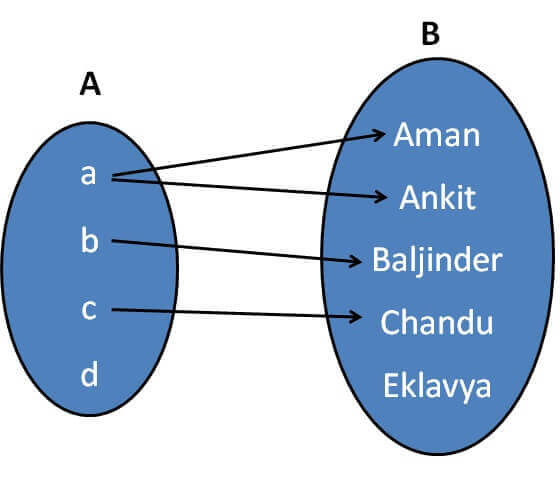Relation is a subset of Cartesian product .

Let us take an example

Let A = {a, b, c, d}

and B = {Aman, Ankit, Baljinder, Chandu, Eklavya}

We define relation as

R = {(x, y): x is the first letter of y, x ∈ A, y ∈ B}

So, our relation R is

R = {(a, Aman), (a, Ankit), (b, Baljinder), (c, Chandu)}

Representing relations with arrow is called arrow diagramNow, in our relation

Domain = Set of all first elements in R

Domain = {a, b, c}

Note that

Domain  ≠ A

Range = Set of all second elements in R

Range = {Aman, Ankit, Baljinder, Chandu}

Codomain = 2nd set

Codomain = Q = {Aman, Ankit, Baljinder, Chandu, Darshan}

1. Chapter 2 Class 11 Relations and Functions (Term 1)
2. Concept wise
3. Relations - Definition

Relations - Definition